# Writing one-step equations word problems

##By Khan Academy

Learn how to write basic equations to model real-world situations.# Writing Equations of Exponential Functions

##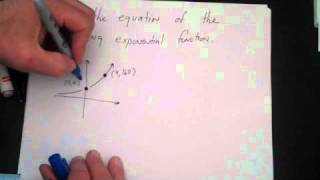By drmikedcook

Writing Equations of Exponential Functions# Writing Equations of Exponential Functions

##By drmikedcook

Writing Equations of Exponential Functions# Writing Equations from a Matrix

##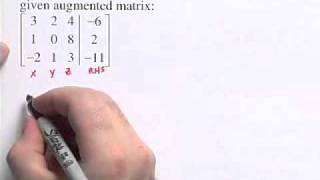By IntFinder

This video explains how to write matrix equations.# Writing The Equation Of A Circle In Standard Form

##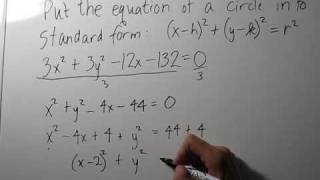By NotesCollegeAlgebra

How to write the equation for the standard form of a circle# Writing proportional equations

##By Khan Academy

Learn how to write a proportional equation y=kx where k is the so-called constant of proportionality.# Writing proportional equations

##By Khan Academy

Learn how to write a proportional equation y=kx where k is the so-called constant of proportionality.# Writing proportional equations

##By Khan Academy

Learn how to write a proportional equation y=kx where k is the so-called constant of proportionality.# Writing proportional equations

##By Khan Academy

Learn how to write a proportional equation y=kx where k is the so-called constant of proportionality.# Writing proportional equations

##By Khan Academy

Learn how to write a proportional equation y=kx where k is the so-called constant of proportionality.# Writing one-step equations word problems

##By Khan Academy

Learn how to write basic equations to model real-world situations.# Writing the equation of a circle when given the center and radius

##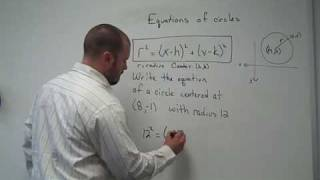By BabelMath

YouTube presents Writing the equation of a circle when given the center and radius, an educational video resource on math.# Writing one-step equations word problems

##By Khan Academy

If you're coming to this video before seeing the previous one, back up! Otherwise, we're solving one-step equations to learn which Super Plan is the best value for our budget.# Writing one-step equations word problems

##By Khan Academy

Using information from the Super Yoga word problem, explore all the possible combinations and create equations which express the possibilities. Let's figure out which plan is best!# Writing Equations of Ellipses In Standard Form and Graphing Ellipses - Conic Sections

##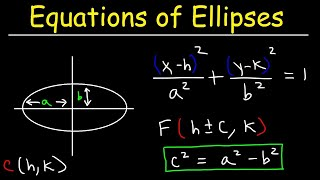By The Organic Chemistry Tutor

This algebra video tutorial explains how to write the equation of an ellipse in standard form as well as how to graph the ellipse when in standard form. It explains how to find the coordinates of the foci, vertices, and co-vertices. This video contains plenty of examples and practice problems.# Equations of conic sections

##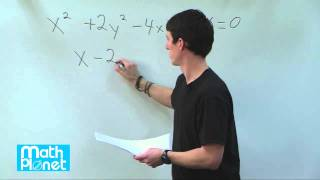By MathPlanetVideos

Write the given equation on the standard equation form for ellipses# Writing Linear Equations | MathHelp.com

##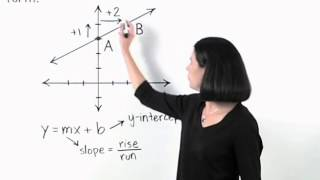By MathHelp.com# Writing the Equation of a Line | MathHelp.com

##By MathHelp.com# Writing systems of equations as matrix equations

##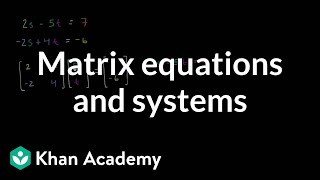By Khan Academy

Sal shows how a system of two linear equations can be represented with the equation A*x=b where A is the coefficient matrix, x is the variable vector, and b is the constant vector.# Writing Equations in Two Variables

##By Anywhere Math